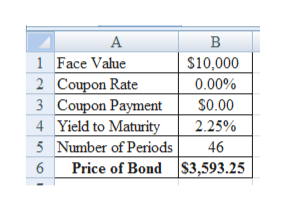You find a zero coupon bond with a par value of \$10,000 and 23 years to maturity. The yield to maturity on this bond is 4.5 percent. Assume semiannual compounding periods. What is the price of the bond?

Question
 You find a zero coupon bond with a par value of \$10,000 and 23 years to maturity. The yield to maturity on this bond is 4.5 percent. Assume semiannual compounding periods. What is the price of the bond?
Step 1

Calculation of Price of Bond:

The price of bond is \$3,593.25.

...help_outlineImage TranscriptioncloseВ 1 Face Value 2 Coupon Rate 3 Coupon Payment 4 Yield to Maturity 5 Number of Periods Price of BondS3,593.25 s10,000 0.00% \$0.00 2.25% 46 fullscreen

Want to see the full answer?

See Solution

Want to see this answer and more?

Our solutions are written by experts, many with advanced degrees, and available 24/7

See Solution
Tagged in